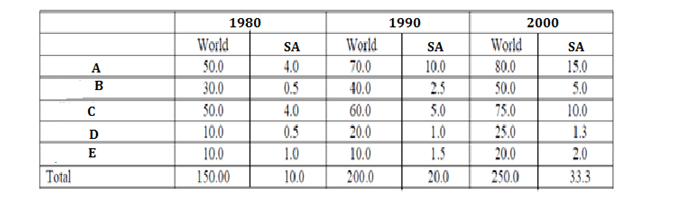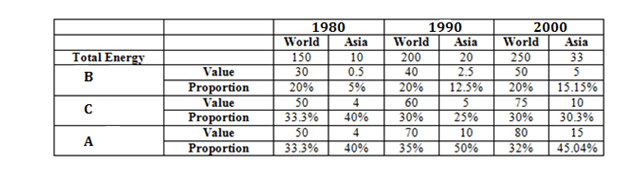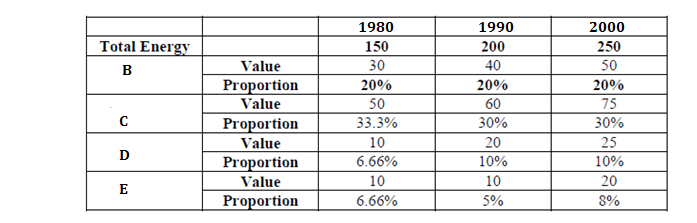Directions: Given below are the forecasts of the World and SA energy demand for the years 1980, 1990 and 2000 AD and the forecast made for these years follows for the subsequent decade as well. For example, the forecast for 1990 means the forecast for years 1990-1999. The demand is given in million barrels per day, crude oil equivalent.Question 1. Over 1990 – 2010, which two fuels meet more than 60 percent of the total energy demand of both World and SA?
(a) A&B
(b) A&C
(c) B&C
(d) None of the above

Question 2. Which fuel’s proportion in the total energy demand increases over the decade 1980–1990 and decreases over the decade 1990 – 2000 for both the World and SA?
(a) A
(b) B
(c) C
(d) D

Question 3. Which is the fuel whose proportion to the total energy demand of the world will remain constant over the period 1980 – 2000 but whose proportion will increase in the total energy demand in SA?
(a) C
(b) D
(c) E
(d) B

Question 4. Which is the fuel whose proportion in the total energy demand will decrease continuously over the period 1980 – 2000, in SA?
(a) B
(b) C
(c) D
(d) E

AnswerThus we can see that C and A combined constitute more than 60% of total energy in both World & SA for the given period.

As seen from the above table, A is the fuel whose proportion in the total energy demand increases during 1980-1990 and decreases during 1990-2000 for both world & SA.

For the answer choices given and for world we can make the following table.